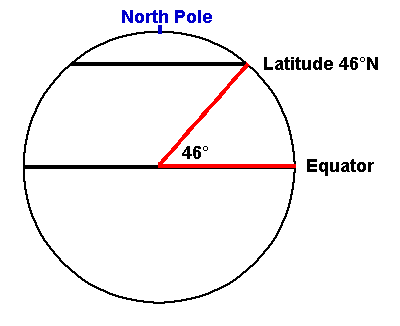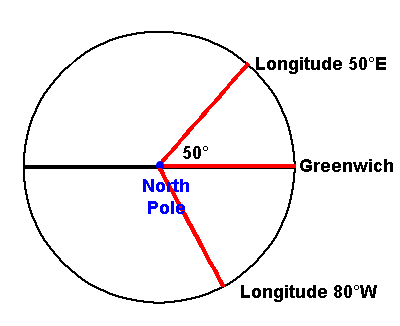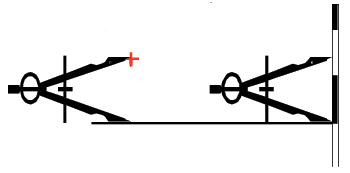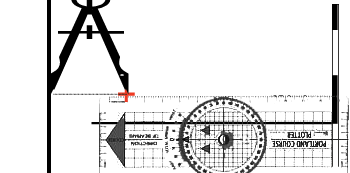# Navigation and Chart work - Latitude and Longitude

##### Latitude

Positions can be given as a latitude and longitude. On Chart 3, on the middle of either side of the chart you will find written 46° 00' north. This line, which forms part of a circle all the way around the World, joins all the points which are 46 degrees north of the Equator (° is the symbol for degrees).Latitude is the angle measured at the centre of the Earth, between the Equator and where you are. It is expressed either north or south, and varies from 0° to 90°.

Each degree is made up of 60 minutes (').

Moving north from 46° on the chart. You can see divisions for 05' and 10' north.

The first of these would be written as 46 ° 05' N and the second 46 ° 10' N

Each of these 05' divisions is made up of 01' divisions. 1' is made up of 60 seconds (''). We do not use seconds of latitude or longitude, as it is far too small a measure for us to draw to.

Each minute is instead divided in to decimals (tenths). On the south east side of the chart, there is a magenta cloured border between Neptunia and New Territories which cuts the side of the chart. This point is 45°47.82'N.

The next border line to the north is at a latitude of 45°56.35'N. Because of the scale of this chart, each minute is broken down in to 5 units of 0.2'.

Different charts have different scales. On Chart 4, the minutes are divided in to 0.1' units. On chart 4F The point on the east side of the chart where the sea changes from white to blue (the 10-metre contour) is at a latitude of 46°16.18'N.

On this chart it is easier to be accurate to two decimal places because the spacing of the markings is further apart.

##### Longitude

Longitude is the angle at centre of the Earth, between where you are and Greenwich. It can be measured either east or west and varies from 0° to 180°.On Chart 3, along the top you will find the 6°00'W point. Travelling west the chart changes from buff to green as you cross the coast at 6° 13.0'W. The 50m depth contour reaches the top of the chart at 6°15.25'W.

On some charts, there may not be a whole number of degrees of longitude because the chart covers too small an area for it to cross the whole degree line, so the meridian (line of longitude) nearest the centre of the chart would have the whole longitude written out, for example 4°35.0'W. Notice that east of this point the next figure is for 4°30.0'W-not 3°30.0'W! This often occurs, if you are not sure of the whole number of degrees on a chart; look at the Southwest or Northeast corner. In very small figures you will find the latitude and longitude of that point, just inside the border.

The latitude and longitude scales are completely different. With your dividers, try measuring 5' of latitude on Chart 3, then compare this to 5' of longitude on the same chart. The latitude is much greater; in fact, the only place where the scales are equal is on the equator.

##### Measuring Latitude and Longitude

To measure the latitude of a feature, use your dividers to measure thedistance between the point, and the nearest line of latitude (horizontal line on the chart), then transfer this distance to the side of the chart, measuring from the same line of latitude.

On Chart 3. What is the latitude of the small dot in the center of the compass rose in the north east corner of the chart?

To find the longitude of a feature, we do a similar thing, but instead measurefrom a line of longitude (a meridian), then transfer to the top or bottom of the chart.

On Chart 3. What is the longitude of the small dot in the center of the compass rose in the north east corner of the chart?

When you have done this a few times you will find that you become quite fast at the process.

Try finding the latitudes and longitudes of the dots in the center of the other compass roses on Chart 1 and Chart 2.

##### Plotting positions on charts

To plot a position on a chart you can not use the dividers to find both distances so we need to use the plotter as a straight edge. By drawing a line out from the side of the chart, at the correct latitude we can then use the dividers to measure off the longitude from a meridian, in the reverse of the operation to find the longitude of a point.

When you do this use the cross lines on the plotter against the gridsdrawn on the chart to ensure you are drawing a line at a right angle to the edge of the chart. Small errors is alignment can produce a large error in the final position.

You could draw a line for both the latitude and longitude but it is more accurate to use the plotter for one and the dividers for the other. Always draw the line that is the shortest distance. On Chart 3, this would often be the line of latitude because of the shape of the chart. Drawing a line in from the sides would probably produce a shorter line than drawing one in from the top or bottom of the chart.

Plot the following positions on Chart 4 and identify the symbol at each point. When you have identified each point accurately draw a small cross at that spot and note the number next to it. You will be able to use them for a later exercise.

1. 46°14.70'N 05°52.25'W

2. 46°15.47'N 05°52.08'W

3. 46°16.08'N 05°49.51'W

4. 46°15.11'N 05°48.83'W